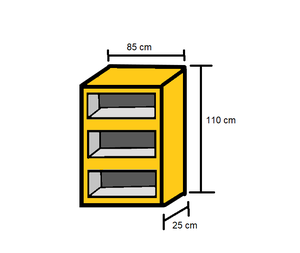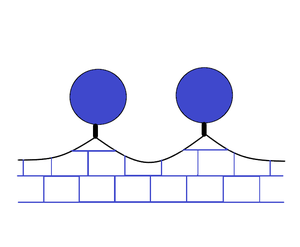Related Articles

# Class 9 NCERT Solutions- Chapter 13 Surface Areas And Volumes – Exercise 13.9

• Last Updated : 28 Dec, 2020

### Question 1: A wooden bookshelf has external dimensions as follows: Height = 110cm, Depth = 25cm, Breadth = 85cm (see fig. 13.31). The thickness of the plank is 5cm everywhere. The external faces are to be polished and the inner faces are to be painted. If the rate of polishing is 20 paise per cm2 and the rate of painting is 10 paise per cm2, find the total expenses required for polishing and painting the surface of the bookshelf.Solution:

Given :External dimensions of book self,

Length, (l) = 85cm

Height, (h) = 110 cm

Formula to calculate external surface area of shelf while leaving out the front face of the shelf = lh+2(lb+bh)

= [85×110+2(85×25+25×110)]

= (9350+9750)

= 19100 cm2

Therefore, the external surface area of shelf is 19100 cm2

Area of front face = [85×110-75×100+2(75×5)]

= 1850+750

= 2600 cm2

Thus, the area is 2600 cm2

Area to be polished surface = (19100+2600) cm2

= 21700 cm2

Given cost of polishing 1 cm2 area = Rs 0.20

Therefore, the cost of polishing 21700 cm2 area Rs. (21700×0.20)

= Rs 4340

Dimensions of the row of the bookshelf

Length, (l) = 75 cm

Height, (h) = 30 cm

Area to be painted in 1 row= 2(l+h)b+lh

= [2(75+30)× 20+75×30]

= (4200+2250)

= 6450 cm2

Thus, the area is 6450 cm2

Area to be painted in 3 rows = (3×6450)cm2

= 19350 cm2.

Given cost of painting 1 cm2 area = Rs. 0.10

Therefore, the cost of painting 19350 cm2 area = Rs (19350 x 0.1) = Rs 1935

Total expense required for polishing and painting = Rs. (4340+1935) = Rs. 6275

Therefore, the cost for polishing and painting the surface of the bookshelf is Rs. 6275.

### Question 2: The front compound wall of a house is decorated by wooden spheres of diameter 21 cm, placed on small supports as shown in fig. 13.32. Eight such spheres are used forth is purpose, and are to be painted silver. Each support is a cylinder of radius 1.5cm and height 7cm and is to be painted black. Find the cost of paint required if silver paint costs 25 paise per cm2 and black paint costs 5 paise per cm2.Solution:

Given: Diameter of wooden sphere = 21 cm

Radius of wooden sphere, r = (21/2) cm = 10.5 cm

Formula for surface area of wooden sphere = 4πr2

= 4×(22/7)×(10.5)2

= 1386 cm3

Thus, the surface area is 1386 cm3

The radius of the circular end of cylindrical support (r) = 1.5 cm

Height of cylindrical support (h) = 7 cm

Formula for curved surface area = 2πrh

= 2×(22/7)×1.5×7

= 66

Thus, the CSA is 66 cm2

The area of the circular end of cylindrical support = πr2

= (22/7)×(1.5)2

= 7.07 cm2

Area of the circular end is 7.07 cm2

Area to be painted silver = [8 ×(1386-7.07)]

= 8×1378.93

= 11031.44 cm2

Area to be painted is 11031.44 cm2

Given cost for painting 1cm2 with silver colour = Rs 0.25

Therefore, the cost for painting 11031.44 cm2 with silver colour = Rs(11031.44×0.25)

=Rs 2757.86

Area to be painted black = (8×66) cm2

= 528 cm2

Given cost for painting 1cm2 with black colour = Rs 0.05

Therefore, the cost for painting with black colour = Rs (528×0.05) = Rs 26.40

Therefore, the total painting cost is:

= Rs (2757.86 + 26.40)

= Rs 2784.26

Hence, the total painting cost will be Rs. 2784.26

### Question 3: The diameter of a sphere is decreased by 25%. By what percent does its curved surface area decrease?

Solution:

Let us assume the diameter of the sphere be “d”.

Radius of sphere, (r1) = d/2

New radius of sphere, (r2) = (d/2)×(1-25/100)

= 3d/8

The curved surface area of sphere, (CSA)1 = 4πr12 = 4π×(d/2)2

= πd2       … (i)

The curved surface area of sphere, (CSA)2 = 4πr22 = 4π×(3d/8)2

= (9/16)πd2     … (ii)

From equation (i) and (ii), we get

Decrease in surface area of sphere will be = (CSA)1 – (CSA)2

= πd2 – (9/16)πd2

= (7/16)πd2

The percentage decrease in surface area of sphere = ((CSA)1 – (CSA)2) / (CSA)1) × 100

= (7πd2/16πd2)×100

= 700/16

= 43.75%

Therefore, the decrease in the percentage of the surface area of sphere is 43.75%

Attention reader! Don’t stop learning now. Join the First-Step-to-DSA Course for Class 9 to 12 students , specifically designed to introduce data structures and algorithms to the class 9 to 12 students

My Personal Notes arrow_drop_up